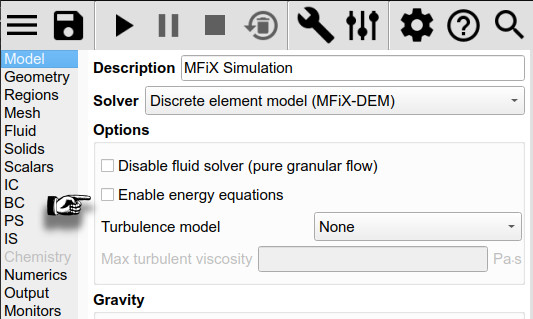# Particle location output

Hi, I have simulate the settling of solid particle under gravity, After it reach a stable fix bed. I want to output the particle location to be used as the particle_input data to simulate the heat and mass transfer further. Thus, may I ask how can I get to location formation of the solid particle in the simulated fixed bed and how to use it as be initial state for particle to further simulate the heat and mass transfer?

Thank you so much for your help

yes i got it. Thank you so much

But i found another problem. I use particle _input_data to generate a random packed bed. However, it seems like it does not run, while no error display.

fluidizedbed.mfx (50.6 KB)
particle_input.dat (311.6 KB)

``````
Program terminated with signal SIGSEGV, Segmentation fault.
#0  0x00007f525655e2b5 in monitor_average_des_mod::monitor_calc_avg_des (lc=4, psize=7161,
at /home/cgw/Work/NETL/mfix/model/monitors/average_des.f90:162
162	          values(lc,1) = values(lc,1) + weight(np)*var(np)
(gdb) p np
\$1 = 49
(gdb) p var
(gdb) up
#1  0x00007f525655f8fe in monitor_functions_des::monitor_collect_data_des (lid=15,
get_value=get_value@entry=
weight=..., vsize=4, values=...)
at /home/cgw/Work/NETL/mfix/model/monitors/functions_des.f90:353
353	         call get_value(lc, psize, mask, weight, vsize, values, des_t_s)
(gdb) p des_t_s
\$3 = <not allocated>
(gdb) quit
``````

This looks like a bug in MFiX. The array `des_t_s` is not allocated, and the monitor code attempts to access this array. We are looking into the problem and will follow up here. Thanks for the report.

– Charles

The reason the array is not allocated is that you have turned off the energy equations.When energy equations are disabled, the particle temperatures are not computed or tracked. The “Temperature” control in the Monitors pane is disabled, as you can see. But the box is checked.

It looks like you must have had energy equations on, enabled the particle temperature monitor, then turned the energy equations off - this results in inconsistent settings and we need to prevent this in the interface.

If you turn energy equations back on, the model should run without problems. If you need to disable energy equations, then turn off the temperature monitors first.

– Charles